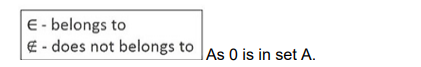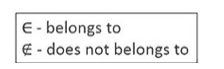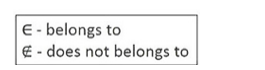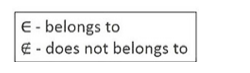# Let A be the set of all even whole numbers less than 10.

Question:

Let A be the set of all even whole numbers less than 10.

(i) Write A in the roster from.

(ii) Fill in the blanks with the approximate symbol ∉ or ϵ :

(a) 0 …. A

(b) 10 …. A

(c) 3 …. A

(d) 6 …. A

Solution:

(i) Whole numbers are 0, 1, 2, 3, …

Even whole numbers less than 10 are 0, 2, 4, 6, 8, 9

So, A = {0, 2, 4, 6, 8}

(ii) (a) Here, A = {0, 2, 4, 6, 8}Hence, 0 ∈ A

(b) Here, A = {0, 2, 4, 6, 8}As 10 is not in a set A

Hence, 10 ∉ A(c) Here, A = {0, 2, 4, 6, 8}

As 3 is not in a set A

Hence, 3 ∉ A

(d) Here, A = {0, 2, 4, 6, 8}As 6 is in set A

Hence, 6 ∈ A